Latest Teaching jobs   »   Mixtures And Allegations- Mathematics Notes For...

# Mixtures And Allegations- Mathematics Notes For CTET Exam: Free PDFMathematics is an equally important section for CTET, MPTET, KVS & DSSSB Exams and has even more abundant importance in some other exams conducted by central or state govt. Generally, there are questions asked related to basic concepts, Facts and Formulae of the Mixtures And Allegations.

To let you make the most of Mathematics section, we are providing important facts related to the Mixtures And Allegations. At least 1-2 questions are asked from this topic in most of the teaching exams. We wish you all the best of luck to come over the fear of the Mathematics section.

How to Overcome Exam Fever, Especially When You Fear Maths

## MIXTURES AND ALLEGATIONS

### MIXTURES

Mixing of two or more qualities of things produces a mixture. When two products of different qualities are mixed, the quality of the resultant mixture lies in between the qualities of the original constituent products, it will be higher than the lowest quality and lower than the highest quality of the items being mixed.

Ex. 17 kg of wheat Atta costing Rs. 29 per kg is mixed with 19 kg Rice Atta costing Rs. 25 per kg. Find the average price of the mixed Atta.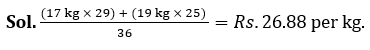If two types of a product of different prices per unit are mixed, the unit price of the resultant mixture will lie between the prices of the two types that form the mixture.

Here, the average quality is essentially the weighted average of the two constituent items.

If q₁ is the quantity or number of items of one particular item of quality P₂, and q₂ be the quantity or number of items of the second item of quality P₂ are mixed together to give a new mixture, then the weighted average value (p) of the quality of the mixture is given by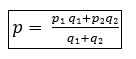Ex. The Ratio of sugar and Salt in 45 litre solution is 5 : 4. How much salt is added to it to make the ratio   10 : 9.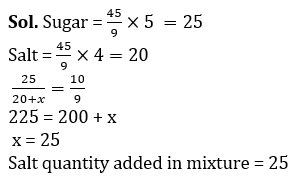If there are more than two groups of items mixed, the weighted average rule can be applied. We will only have to take figures (as shown in the formula for the two groups) for all the groups in the numerator as well as the denominator and calculate the weighted average. For example, if there are four groups of quantities q₁, q₂, q₃ and q₄ whose respective qualities are p₁, p₂, p₃ and p₄, then the weighted average quality of the group can be written as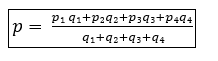A mixture can also be a solution – that is, a liquid mixed with another liquid which is normally water. The concentration of the solution is expressed as the proportion or percentage of the liquid in the total solution.

For example, if 20 litres of pure alcohol is mixed with 60 litres of water, then in a total solution of 80 litres, there is 20 litres of alcohol. So, concentration of this solution is 0.25 (= 20/80) or 25%.

Similarly, if 30 litres of pure milk is mixed with 10 litres of water, the concentration of this solution can be expressed as 75% (= 30/40) milk or 25% water.

We can also have two solutions mixed together to give a new solution. Such problems can also be handled in the same manner as other mixtures. In the weighted average rule, the quality of the constituents (p₁, p₂, etc.) will then be the concentrations of various solutions mixed together.

Ex. In an examination consisting of eight subjects Rohit scored 85, 85, 90, 91, 92 94, 95 and 96 marks. Find his average score.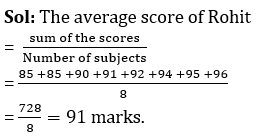Alternate Method:

The problem. can be solved using assumed average/mean also.

Let us take 90 as the assumed mean, then the actual average score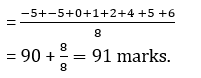### ALLEGATIONS

We will take the Weighted Average rule discussed in the previous section and rewrite the formula such that the quantity terms come on one side and the price terms come on the other side. If we do this, we get the rule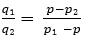This is called the RULE OF ALLEGATION. This rule connects quantities and prices in mixtures. This can also be written as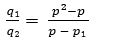In a descriptive manner, the Rule of Allegation can be written as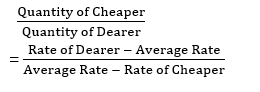The above formula can be represented as follows: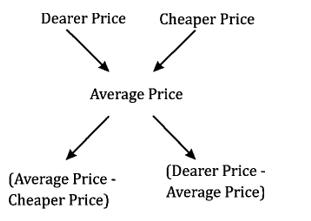Ex. A shopkeeper has 150 kg of wheat, He sell one part of it at 18% profit and rest at 9% loss. On the whole sale his loss is 3%. Find the Quantity of Wheat sold at 9% loss?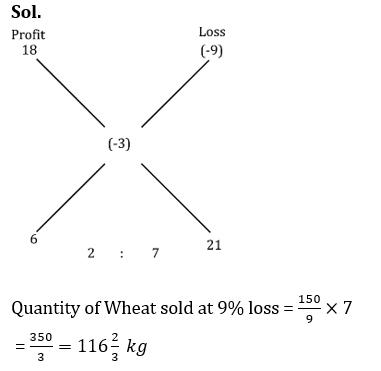If there is P volume of pure liquid initially and in each operation, Q volume is taken out and replaced by Q volume of water, then at the end of n such operations, the concentration (k) of the liquid in the solution is given by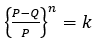This gives the concentration (k) of the liquid as a PROPORTION of the total volume of the solution.” If the concentration has to be expressed as a percentage, then it will be equal to 100k. If the volume of the liquid is to be found out at the end of n operations, it is given by KP. i.e., the concentration k multiplied by the total volume P of the solution.

Ex. An alloy contains Iron, Copper and Bronze in the ratio of 4 : 3 : 2. The quantity of Bronze that must be added to 99 kg of this alloy to have the new ratio 4 : 3 : 3  is

Sol.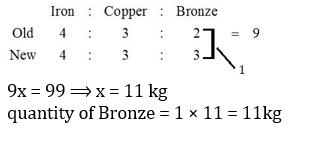•विशेषण- परिभाष�...
•Phases of Teaching - Stages of Teaching ...
•Operant and Classical Conditioning - Dif...
•संज्ञा: परिभाष�...
•अलंकार - परिभाष�...
•Stages of Development- Child Growth and ...